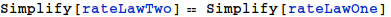Derivation of the Rate Law for the the Reversible Michaelis-Menten Mechanism

Created with Wolfram Mathematica

1. Preliminaries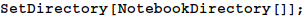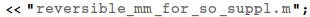2. Mechanism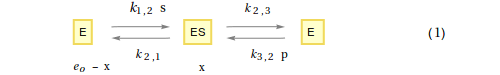3. Derivation of the rate-constant form of the Rate Law

Set up the differential equation

Let $x$ be the conentration of ES

Let $e_o$ be the total enzyme concentration

Therefore, the concentration of E (the 'free' enzyme concentration) equals $e_o$ - $x$

From the steady-state assumption, the rate of formation of x will equal the rate of breakdown of $x$, and the following differential equation may be written

$${dx\over dt} = {k_{1,2}\ (e_o -x)\ s + k_{3,2}\ (e_o -x)\ p - (k_{2,1} + k_{2,3})\ x = 0}\ \ \ \ \ \ \ (2)$$

Solving for $x$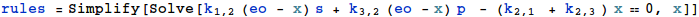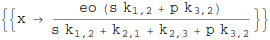4. Velocity equation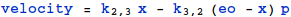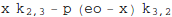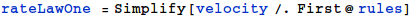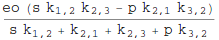5. Define Kinetic Constants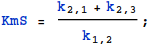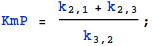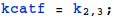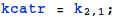6. The Kinetic-Constant form of the Rate  Law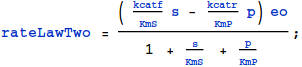7. An Important Check